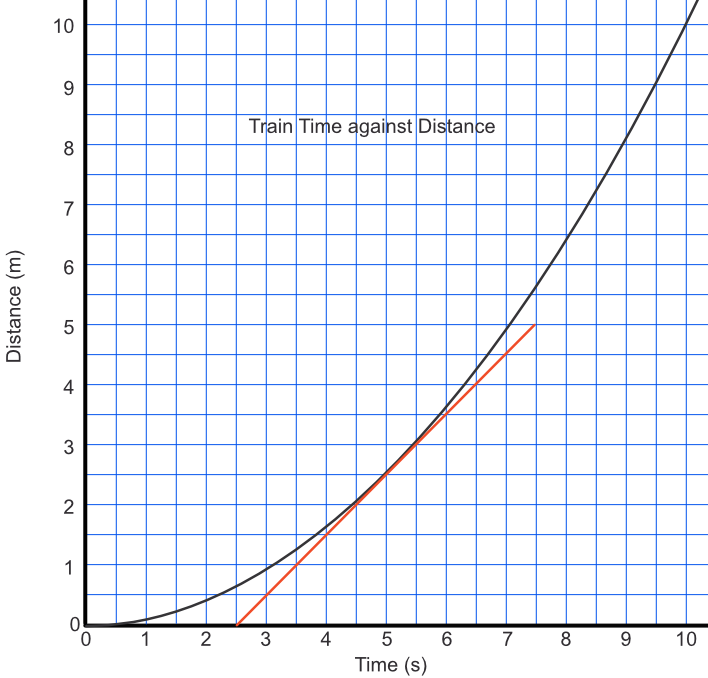With a curved graph the gradient changes continuously. The gradient gives the rate of change of the item being measured. For kinematic (speed) graphs, the rate of change on a distance-time graph gives velocity. On a velocity-time graph, the rate of change gives acceleration.

To estimate the gradient of a curved line, draw the graph accurately. Then draw a tangent to the graph at the point at which the gradient is required.

## Example 1

A train moves away from a station with an acceleration of 0.2 ms-2. By drawing a graph of time against distance, estimate the speed of the train after 5 seconds.

The distance travelled by the train is given by s = frac(1)(2)at^2, where s is distance, t is time and a is acceleration.

The velocity of the train is given by the gradient of the graph at the point in time in question.

Take the tangent to the graph at x = 5. Extend the line to measure a reasonable gradient. Gradient measures as frac(5-0)(7.5-2.5) = frac(5)(5) = 1.

 time (s) 0 2 4 6 8 10 dist (m) 0 0.4 1.6 3.6 6.4 10